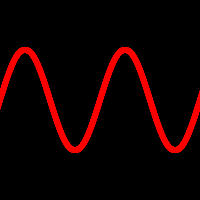# waveField

field
waveField
RayTK
v0.36

A field that uses a periodic wave.

If there is an input, that rop is used to get the coordinate that is fed into the wave function. Without an input, the Axis is used to run the wave function on the position along the selected axis.

## Parameters

• `Enable`:
• `Wave`: The type of wave.
• `Sine`:
• `Cosine`:
• `Triangle`:
• `Ramp`:
• `Reverse Ramp`:
• `Square`:
• `Additive Square (2)`:
• `Additive Square (4)`:
• `Additive Square (8)`:
• `Axis`: Axis used for the wave function phase. If there is a coordinate field, this controls which part of the returned value is used. Otherwise it controls which part of the position in used.
• `X`:
• `Y`:
• `Z`:
• `Distance From Origin`: Uses distance from the origin instead of an axis.
• `Coord Type`:
• `Auto`:
• `1D`:
• `2D`:
• `3D`:
• `Period`: The length of a single cycle of the wave.
• `Phase`: Offset of the wave along the axis / coordinates.
• `Amplitude`: The height of the wave, which scales the range of output values. If this is set to 3 (and `Offset` is 0), a ramp wave will produce values from 0 to 3.
• `Offset`: Adds to the values produced by the wave. If this is set to 0.5 (and `Amplitude` is set to 1), a ramp wave will produce values from 0.5 to 1.5.
• :

## Inputs

• `Coordinate Field`: (optional) If attached, the wave will use this to determine the numbers that it passes to the wave function (instead of using the position along the chosen `Axis`).
• Coordinate Types: `float` `vec2` `vec3` `vec4`
• Context Types: `Context` `MaterialContext` `CameraContext` `LightContext` `RayContext` `ParticleContext`
• Return Types: `float` `vec4`
• `Period Field`: (optional) If attached, the wave will use this field to multiply the `Period` parameter, which can be used for frequency modulation.
• Coordinate Types: `float` `vec2` `vec3` `vec4`
• Context Types: `Context` `MaterialContext` `CameraContext` `LightContext` `RayContext` `ParticleContext`
• Return Types: `float`
• `Phase Field`: (optional)
• Coordinate Types: `float` `vec2` `vec3` `vec4`
• Context Types: `Context` `MaterialContext` `CameraContext` `LightContext` `RayContext` `ParticleContext`
• Return Types: `float`
• `Wave Function`: (optional)
• Coordinate Types: `float`
• Context Types: `Context` `MaterialContext` `CameraContext` `LightContext` `RayContext` `ParticleContext`
• Return Types: `float` `vec4`
• `Amplitude Field`: (optional)
• Coordinate Types: `float` `vec2` `vec3` `vec4`
• Context Types: `Context` `MaterialContext` `CameraContext` `LightContext` `RayContext` `ParticleContext`
• Return Types: `float`